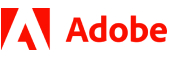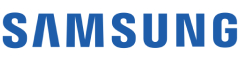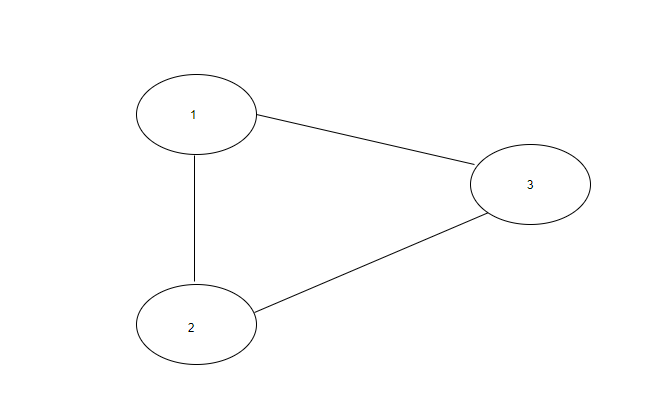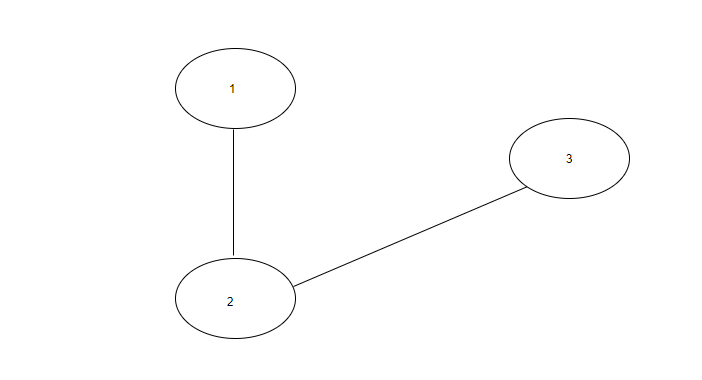New update is available. Click here to update.
Topics

# Cycle Detection In Undirected Graph

Moderate0/80+14 more companies

## Problem statement

You have been given an undirected graph with 'N' vertices and 'M' edges. The vertices are labelled from 1 to 'N'.

Your task is to find if the graph contains a cycle or not.

A path that starts from a given vertex and ends at the same vertex traversing the edges only once is called a cycle.

Example :

``````In the below graph, there exists a cycle between vertex 1, 2 and 3.
``````Note:

``````1. There are no parallel edges between two vertices.

2. There are no self-loops(an edge connecting the vertex to itself) in the graph.

3. The graph can be disconnected.
``````

For Example :

``````Input: N = 3 , Edges =  [[1, 2], [2, 3], [1, 3]].
Output: Yes

Explanation : There are a total of 3 vertices in the graph. There is an edge between vertex 1 and 2, vertex 2 and 3 and vertex 1 and 3. So, there exists a cycle in the graph.
``````
Detailed explanation ( Input/output format, Notes, Images )
Constraints:
``````1 <= T <= 10
1 <= N <= 5000
0 <= M <= min(5000, (N * (N - 1)) / 2)
1 <= edges[i] <= N
1 <= edges[i] <= N

Time Limit: 1 sec
``````
Sample Input 1:
``````1
3 2
1 2
2 3
``````
Sample output 1:
``````No
``````
Explanation of Sample output 1:
`````` The above graph can be represented as
````````````There are a total of 3 vertices in the graph.There is an edge between vertex 1 and 2 and vertex 2 and 3. So, there is no cycle present in the graph.
``````
Sample Input 2:
``````2
4 0
4 3
1 4
4 3
3 1
``````
Sample output 2:
``````No
Yes
``````Console